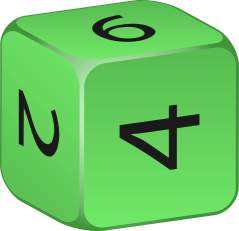# 6 Sided Dice Probability Calculator

Created by Wojciech Sas, PhD candidate
Reviewed by Arturo Barrantes
Last updated: Aug 30, 2022

If you ever wondered how to calculate different probabilities using typical six-sided dice, here is Omni's 6 sided dice probability calculator to dispel any doubts!

Using our tool, you'll find answers to the following questions:

• How to calculate probabilities for a six-sided dice set?
• What is 2d6 probability?
• What is the most likely sum of rolling three dice?

And many more. Let's roll a die!

## What is a 6-sided die?

A six-sided die is the standard die with a cubic shape. Each face has a different value, typically from 1 to 6. A fair 6-sided die gives you a 1/6 (or ca. 16.7%) probability of rolling any of its numbers.

## How to use 6 sided dice probability calculator?

To find any of the probabilities with our 6-sided dice probability calculator, follow these steps:

1. Select the number of dice you want to roll.
2. Choose the game condition. You can switch between rolling a specific value, checking if the sum of all dice is equal/higher/smaller than a given target value, or a few others.
3. For some options, you may need to type which face numbers meet the winning conditions.
4. That's all! The resulting probability will display at the bottom.

If you enjoy reading about numerous dice probabilities, you may find our other tools handy:

## FAQ

### How do I calculate the probability of rolling 4 or higher with three dice?

To find the probability of rolling 4 or higher using three 6-sided dice:

1. Estimate the wanted probability for a single die. Three results succeed (4, 5, 6), and three fail (1, 2, 3), so the probability for a single die is 1/2.
2. Multiply the auxiliary probabilities (each for a separate die): 1/2 × 1/2 × 1/2.
3. The result equals 1/8 or 12.5%.

### What does 2d6 probability mean?

2d6 stands for rolling two cubic (6-sided) dice. We usually use this setup to get the sum from both dice. The most likely sum is 7 (the probability equals 16.7%). The least likely sums are 2 or 12 (2.78% each).

### What is the most likely sum of rolling three dice?

10 or 11. Out of the total of 216 rolling possibilities (permutations with repetitions), there are precisely 57 sets that sum up to 10 and the same number which sum up to 11. The probability of rolling 10 or 11 as the sum of three dice is 12.5% (for each option).

Wojciech Sas, PhD candidate
Choose the number of diceTotal number of dice
Choose the conditions - Game rules
I want to get:
All dice with the same face/side value
The dice value I want to get:
...which results in...
The probability of getting any value from the dice
People also viewed…

### 99% confidence interval

The 99% confidence interval calculator will help you with the fundamental statistics problem of estimating a specific confidence interval.

### Alien civilization

The alien civilization calculator explores the existence of extraterrestrial civilizations by comparing two models: the Drake equation and the Astrobiological Copernican Limits👽

### Books vs e-Books

The books vs. e-books calculator answers the question: how ecological is your e-book reader? 📚

### Random number

No more straining with the tossing a coin and the rolling of dice - this random number generator will give you the figures you need.